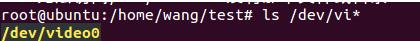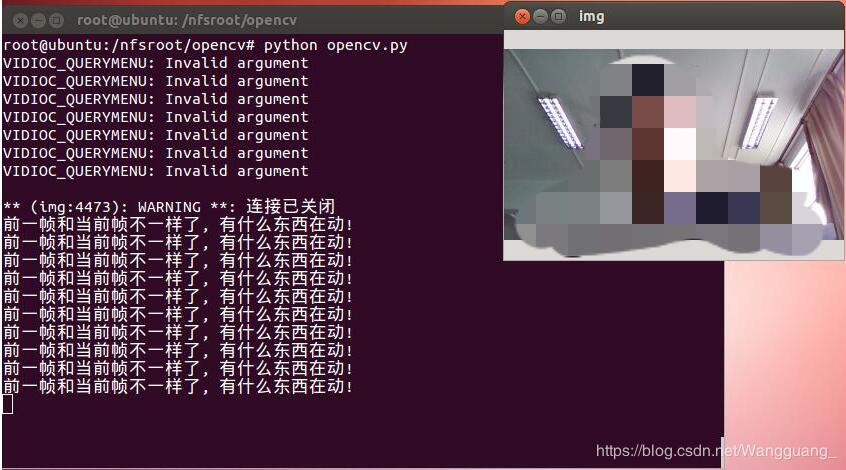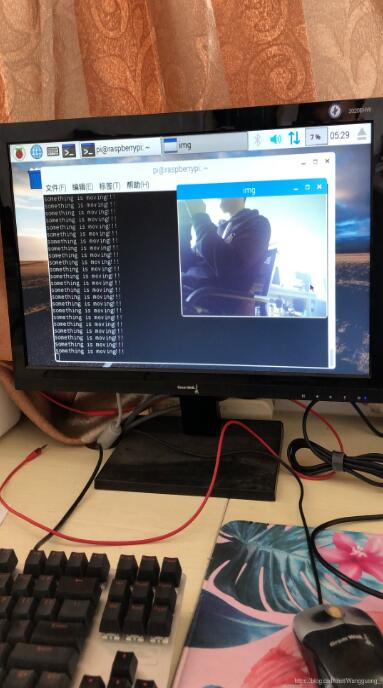# 树莓派+摄像头实现对移动物体的检测

更新时间：2019年06月22日 09:56:37   作者：Wang哈哈我要评论

`ls /dev/video*````#encoding=utf-8
import RPi.GPIO as GPIO
import cv2
import time
import os

GPIO.setmode(GPIO.BCM)
GPIO.setup(18,GPIO.OUT)

camera = cv2.VideoCapture(0)
if camera is None:
print('please connect the camera')
exit()

fps = 30
pre_frame = None

led = False

while True:
start = time.time()
res, cur_frame = camera.read()
if res != True:
break
end = time.time()
seconds = end - start
if seconds < 1.0/fps:
time.sleep(1.0/fps - seconds)

cv2.namedWindow('img',0);
#cv2.imshow('img', cur_frame)
key = cv2.waitKey(30) & 0xff
if key == 27:
break

gray_img = cv2.cvtColor(cur_frame, cv2.COLOR_BGR2GRAY)
gray_img = cv2.resize(gray_img, (500, 500))
gray_img = cv2.GaussianBlur(gray_img, (21, 21), 0)

if pre_frame is None:
pre_frame = gray_img
else:
img_delta = cv2.absdiff(pre_frame, gray_img)
thresh = cv2.threshold(img_delta, 25, 255, cv2.THRESH_BINARY)
thresh = cv2.dilate(thresh, None, iterations=2)

contours, hierarchy = cv2.findContours(thresh.copy(),cv2.RETR_LIST,cv2.CHAIN_APPROX_SIMPLE)
for c in contours:
if cv2.contourArea(c) < 1000:
continue
else:
(x,y,w,h) = cv2.boundingRect(c)
cv2.rectangle(cur_frame,(x,y),(x+w,y+h),(0,255,0),2)

print("something is moving!!!")
led = True
if led == True:
for i in range(30):
GPIO.output(18,GPIO.HIGH)
time.sleep(0.03)
GPIO.output(18,GPIO.LOW)
time.sleep(0.03)
break

cv2.imshow('img', cur_frame)
pre_frame = gray_img

camera.release()
cv2.destroyAllWindows()```

Ubuntu下的运行结果如下